Short articles on topics in systematic trading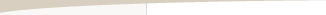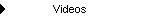Other Articles...

Position sizing optimizationPosition Sizing Optimization

by Michael R. Bryant

Position sizing is the process of determining the number of shares or contracts to trade. Two of the more popular methods are fixed fractional and fixed ratio position sizing. Both of these methods have an adjustable parameter that determines how quickly contracts or shares are added as the account equity grows or trading profits accumulate. A suitable value for the position sizing parameter -- for example, the delta of fixed ratio position sizing -- isn't always obvious. Position sizing optimization is a way to find the optimal settings for any position sizing method.

Optimization involves goals or objectives, constraints, and parameters. The purpose of the optimization is to find the value of the parameter that minimizes or maximizes the objective without violating the constraints. The result of this process is the optimal value of the parameter. A search method is used to find the optimal parameter value.

As an example, suppose we want to maximize the rate of return in fixed ratio position sizing without exceeding a worst-case drawdown of 30%. Our objective is the rate of return. The constraint is the limit on the worst-case drawdown to no more than 30%. The optimization parameter is the delta of the fixed ratio method. The search method simulates trading using different delta values, searching for the delta that generates the highest return without exceeding the drawdown constraint. The solution is the optimal delta.

For position sizing optimization, almost any performance measure can be used as an objective. Some common objectives might be net profit, rate of return, average trade, profit factor, return-drawdown ratio, and Sharpe ratio. Alternatively, any of these could be used as a constraint. A particularly useful constraint is the maximum (worst-case) percentage drawdown, defined as the largest percentage decline from a prior peak in equity. Most traders have some idea of what kind of drawdown they can tolerate; for example, a 20% drop in equity. Applying this limit as an optimization constraint insures that the optimal result won't exceed this threshold.

When optimizing trading system results, it's important to remember that the past won't ever repeat exactly. While the overall distribution of profits and losses (that is, the numbers and sizes of the profits and losses) may remain more or less the same in the future, the sequence of those trades will probably be much different in the future. In particular, the worst-case drawdown is heavily dependent on the sequence of trades. If you optimize over the historical sequence of trades assuming that the worst-case drawdown will be no worse in the future, you could be end up risking too much on each trade.

One way to avoid this problem is to optimize using Monte Carlo analysis. Briefly (click here for more on Monte Carlo analysis), Monte Carlo analysis allows you to introduce randomness into the simulation of the equity curve. Instead of duplicating the historical sequence of trades, the trade sequence is randomized, and the results for the random sequence are tabulated over hundreds or thousands of repetitions. This process tends to generate a more conservative position sizing result, which makes it less likely that you'll risk too much.

The other way to account for the changing nature of the markets is to save a portion of the data for out-of-sample testing. For example, suppose you have a series of 500 trades. You might perform position sizing optimization on the first 300 trades, then test the optimal results on the remaining 200 trades. Because the optimization process didn't use the second set of 200 trades, testing the optimal results on this set of trades will give you a better idea of what to expect in the future.

A formal method of out-of-sample testing is walk-forward testing or walk-forward optimization. In walk-forward optimization, the optimization is performed on a sliding window of data. After the results are obtained for one window, they're tested forward on out-of-sample data. The window is moved forward, the optimization is repeated, and the results for the new window of data are tested forward. This process is repeated until there are no more data to test.

For example, to perform position sizing optimization in a walk-forward manner, you might optimize over trades 1 - 50 (window #1), then test the optimal result on trade 51 (the out-of-sample test). Then optimize over trades 2 - 51 and test the optimal parameter on trade 52. The third optimization window would be trades 3 - 52, followed by the third out-of-sample test on trade 53, and so on. The results of the optimization are judged based on the out-of-sample test results.

An example of position sizing optimization is shown below. The fixed fraction was optimized by maximizing the net profit over the existing sequence of trades with a drawdown constraint of 25%. The optimal fixed fraction was found to be 4.00%.Example of optimal position sizing results.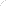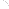Join Our Email List Email: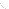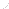For Email Marketing you can trust